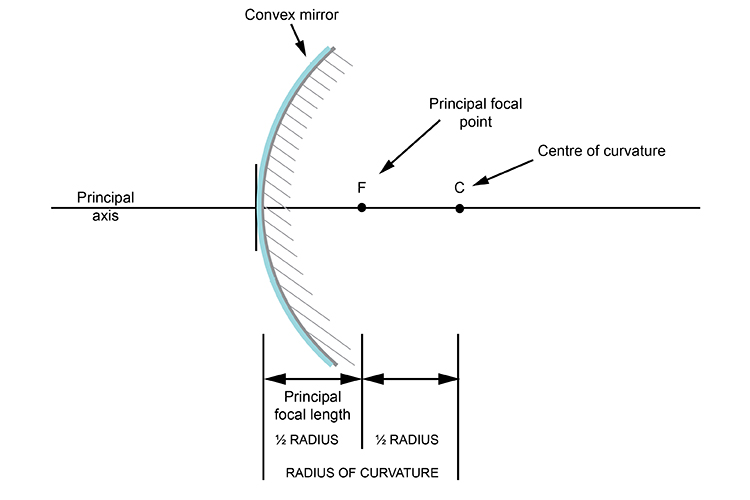# Centre of curvature – Convex mirrors

Unlike lenses the rays bouncing off the convex mirror are entirely dependent on the centre of curvature. This is because the principal focal length is half the radius of curvature of the convex mirror.This would translate mathematically as.

Pr\i\n\c\i\p\a\l\ \f\o\c\a\l\ \l\e\n\g\t\h = (ra\d\i\u\s\ \c\u\r\v\a\t\u\r\e)/2

or

Pr\i\n\c\i\p\a\l\ \f\o\c\a\l\ \l\e\n\g\t\h = R/2

We must emphasise again that this is only true of convex mirrors and not convex lenses.